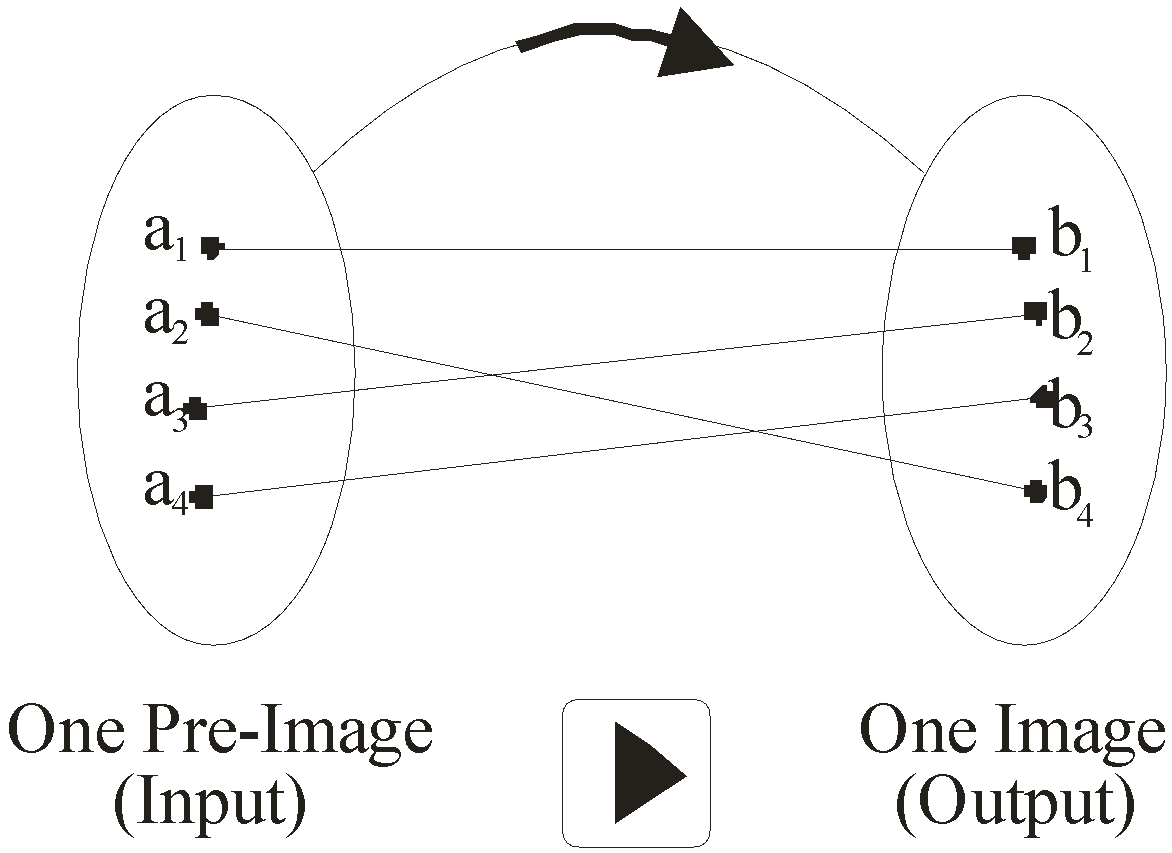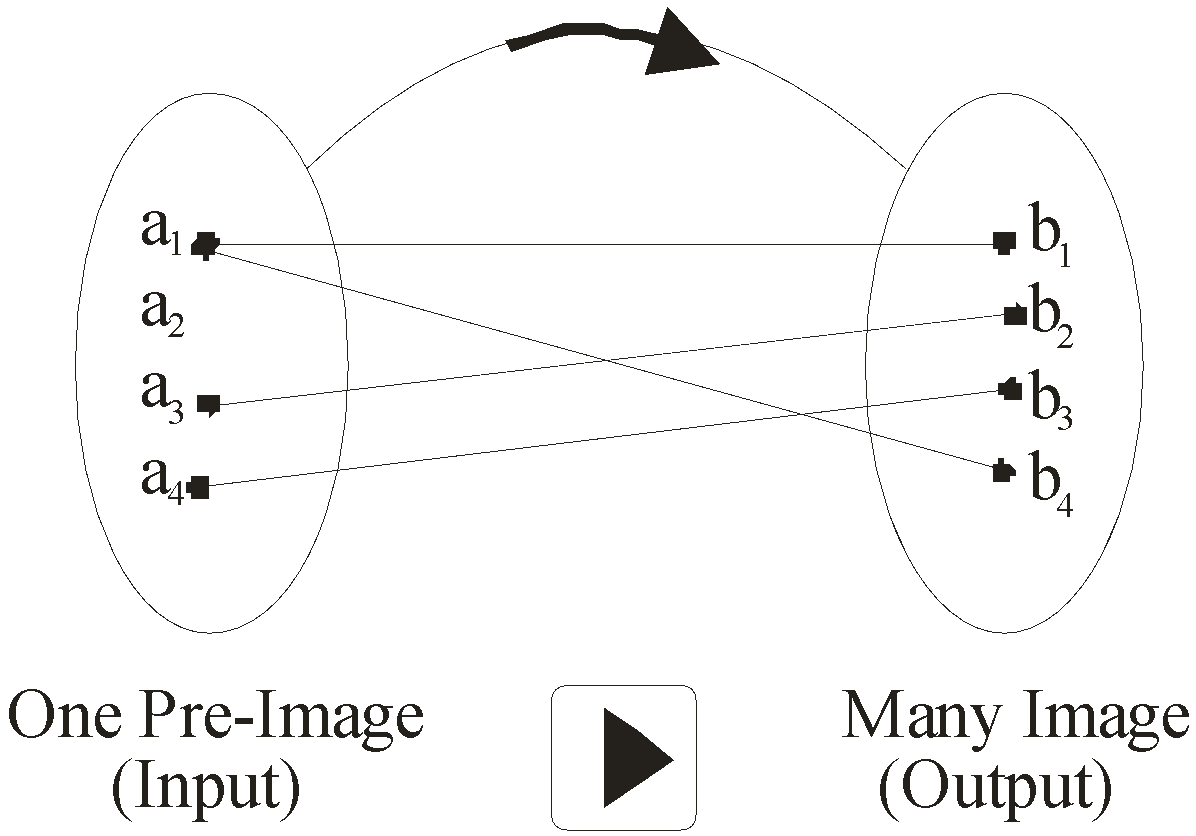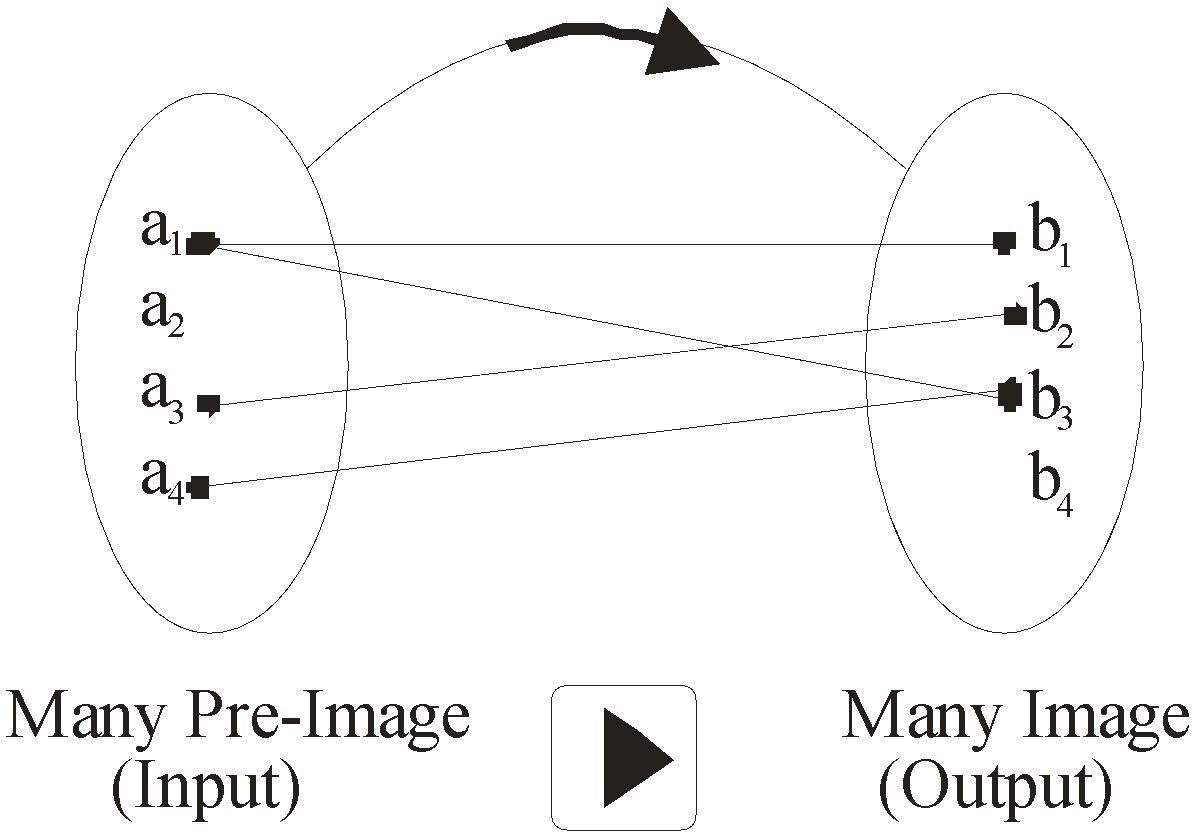BodhiAI
Nov. 11, 2019

#### Algebra of Function, Types of Function(one to one,one to many):

Types of Correspondence

A. One-One Correspondence

If to every element of set A, there corresponds one and only one element of set B, then the correspondence from A to B is one-to-one correspondence.B. Many-One Correspondence

If to more than one element of set A, then corresponds onsly one element of set B, then the correspondence from A to B is Many-to-one correspondence.C. One-Many Correspondence

If to at least one element of set A, there corresponds two or more elements of set B, then the correspondence from A to B is called one-to-many correspondence.D. Many-Many Correspondence

If a correspondence is both one-to-many as well as many-to-one then it s called Many-Many correspondence .